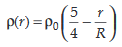# Let there be a spherically symmetric charge distribution with charge density varying as upto r = R, and r(r) = 0 for r > R, where r is th...

Let there be a spherically symmetric charge distribution with charge density varying asupto r = R, and r(r) = 0 for r > R, where r is the distance from the origin. The electric field at a distance r (r < R) from the origin is given by

Anonymous User Physics Electrostatic 11 May, 2020 54 views